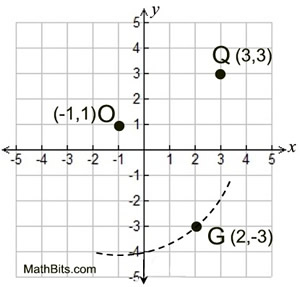Proofs in Coordinate Geometry Terms of Use    Contact Person: Donna RobertsDirections: Prepare a coordinate geometry proof for each problem. Some problems specify a method, while others leave the choice of method up to you. While more than one method of proof, or presentation, is possible, only one possible answer will be shown for each question.

 1 Given: quadrilateral PQRS with P(-4,-2), Q(-2,3), R(4,3) and S(2,-2). Prove: PQRS is a parallelogram using slopes.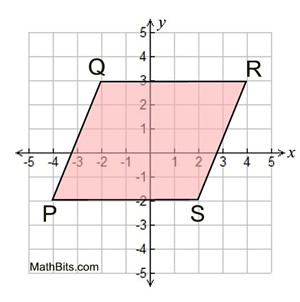2 Given: ΔDOG with D(-2,5), O(-4,1) and G(-10,4) Show: ΔDOG is a right triangle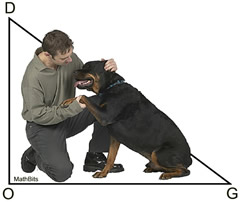3 Given: quadrilateral FRED with F(0,3), R(4,5), E(5,3) and D(1,1) Prove: FRED is a rectangle4 Given: quadrilateral ABCD with A(5,1), B(0,3), C(-2,5) and D(3,3) Show: ABCD is a parallelogram using midpoints.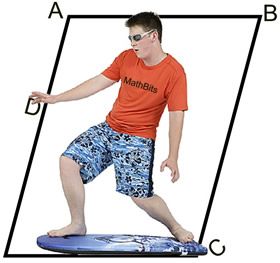5 Given: quadrilateral CDEF with C(-2,3), D(-5,-4), E(2,-1) and F(5,6) Prove: CDEF is a rhombus but not a square6 Given: ΔFUN with F(4,-1), U(5,6) and N(1,3) Show: ΔFUN is an isosceles right triangle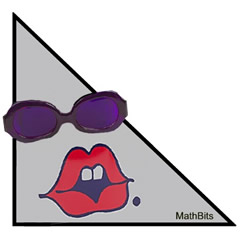7 Given: quadrilateral TRAP with T(-5,7), R(-3,-4), A(9,5) and P(-1,10) Show: TRAP is an isosceles trapezoid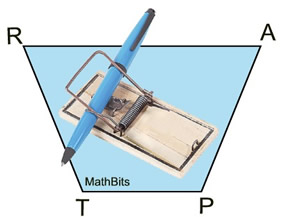8 Given: quadrilateral ARMY with A(p,q), R(0,0), M(r,0) and Y(p+r,q) Prove ARMY is a parallelogram.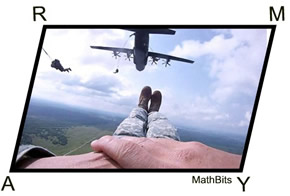9 Given: ΔPQR with P(-1,4), Q(3,0), and R(-2,-3) Prove ΔPQR is NOT a right triangle.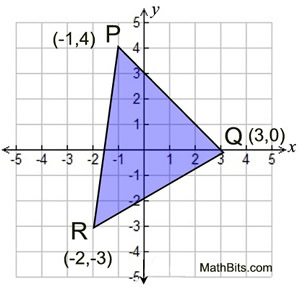10 Given: Circle O with center at (-1,1) and point G(2,-3) lies on the circle. Create a proof to show whether point Q(3,3) lies on the circle, or not.